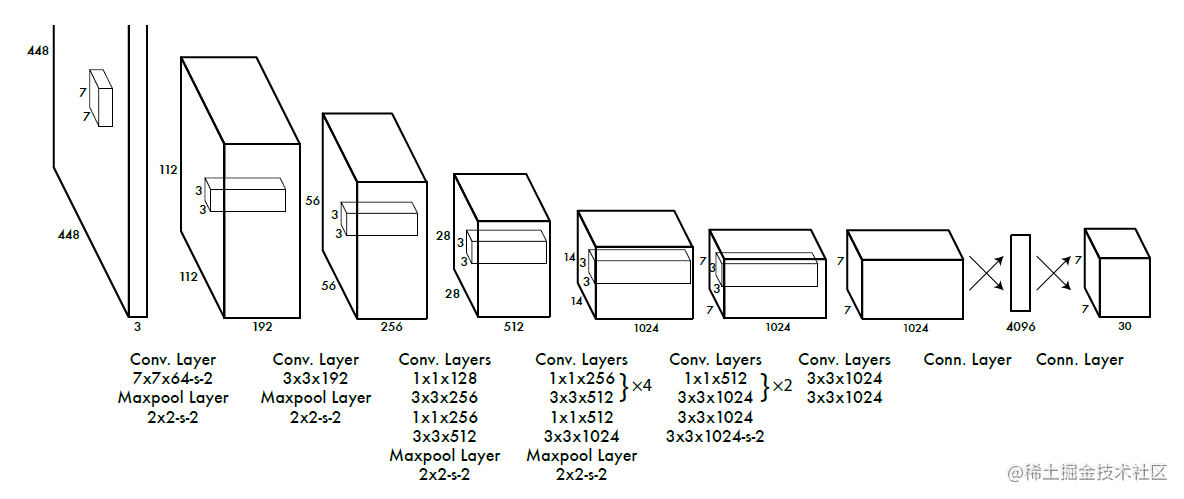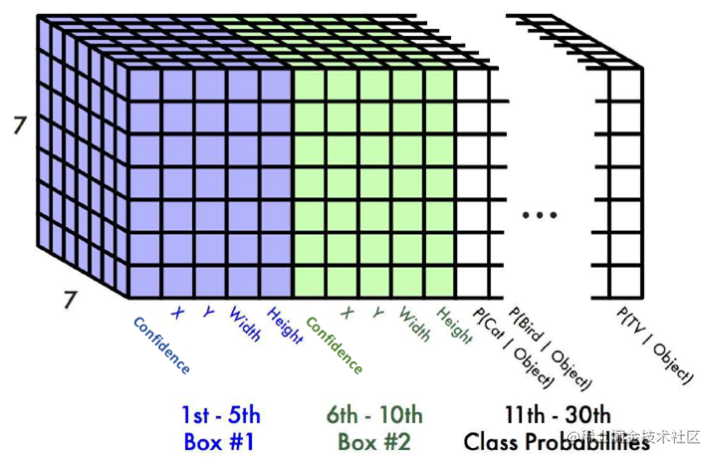# 虽然有些过时，但如果自己动手实现一遍 YOLOv1 势必会有所收获(1) 网络架构### 动机### 代码实现

YOLOv1 使用 Darknet 开发的，这是比较灵活用于研究深度学习框架，最近自己也在尝试写深度学习网络框架，不为别的，就是为了让自己能够更深入熟悉神经网络而已。回到 Darknet 这是一个比较底层 c 语言开发框架，对于自己来说最熟悉还是 pytorch 所以当然首先 pytorch 来实现。

### 依赖

• torch
• numpy

import torch
import torch.nn as nn

### 网络结构

#### 配置文件设计

• 字符串类型: 表示池化层也就是非卷积层的层，例如 "M" 当然这里也只出现了 "M" 表示 Maxpooling
• 列表类型: 表示一组可重复的由多个卷积块组成模块，例如 [(1,256,1,0),(3,512,1,1),4], 其中 4 表示可重复模块，(1,256,1,0),(3,512,1,1)network_config = [
"M",#maxpooling
(3,192,1,1),# 2
"M",
(1,128,1,0),#1x1 kernel 用于改变通道数 3
(3,256,1,1),#4
(1,256,1,0),#5
(3,512,1,1),#6
"M",
[(1,256,1,0),(3,512,1,1),4],#14 list 表示重复块
(1,512,1,0),#15
(3,1024,1,1),#16
"M",
[(1,512,1,0),(3,1024,1,1),2],#17
(3,1024,1,1),#18
(3,1024,2,1),#19
(3,1024,1,1),#20
(3,1024,1,1),#21
]#### CNNBlock 结构

CNNBlock 是网络的基础结构，输入特征图经过卷积层后进行标准化(batchNorm)，这里有点不同于原论文，因为当时 YOLOv1 并没有采用 BN 层，这个应该是在 YOLOv2 中采取的优化内容，然后进行激活层，这里激活函数采用 leakyRule 这个激活函数。

class CNNBlock(nn.Module):
def __init__(self, in_channels, out_channels,**kwargs):
super(CNNBlock,self).__init__()

self.conv = nn.Conv2d(in_channels,out_channels,bias=False,**kwargs)
self.batchnorm = nn.BatchNorm2d(out_channels)
self.leakyrelu = nn.LeakyReLU(0.1)

• 因为采用了 BN 层，所以在卷积层将bias=False ，关于各个层的命名这里并没有采取驼峰命名规则，而是一路小写，主要目的是为了便于和 torch 的 API 进行适当的区别。class CNNBlock(nn.Module):
def __init__(self,in_channels,out_channels,**kwargs):
super(CNNBlock,self).__init__()
self.conv = nn.Conv2d(in_channels,out_channels,bias=False,**kwargs)
self.batchnorm = nn.BatchNorm2d(out_channels)
self.leakyReLU = nn.LeakyReLU(0.1)

def forward(self,x):
x = self.conv(x)
x = self.batchnorm(x)
x = self.leakyReLU(x)
return x

class Yolov1(nn.Module):
def __init__(self,in_channels=3,**kwargs):
super(Yolov1,self).__init__()

self.config = network_config
self.in_channels = in_channels
self.featureextractor = self._create_conv_layers(self.config)

def forward(self, x):
x = self.featureextractor(x)

def _create_conv_layers(self,config):
layers = []
in_channels = self.in_channels

for module in config:
if isinstance(module,tuple):
layers += [CNNBlock(in_channels,
module,
kernel_size=module,
stride=module,
in_channels = module
elif isinstance(module,str):
layers += [nn.MaxPool2d(kernel_size=(2,2),stride=(2,2))]
elif isinstance(module,list):
conv1 = module
conv2 = module
num_repeats = module
for _ in range(num_repeats):
layers += [
CNNBlock(in_channels,
conv1,
kernel_size=conv1,
stride=conv1,
]

layers += [
CNNBlock(conv1,
conv2,
kernel_size=conv2,
stride=conv2,
]

in_channels = conv2

return nn.Sequential(*layers)
def _create_fcs(self,split_size,num_boxes,num_classes):
S, B, C = split_size,num_boxes,num_classes
return nn.Sequential(
nn.Flatten(),
nn.Linear(1024 * S * S,496), # 4096
nn.Dropout(0.0),
nn.LeakyReLU(0,1),
nn.Linear(496,S*S*(C + B*5))
)

YOLOv1 结构中主要包括两个部分一个提取特征的卷积结构，一部分是作为进行分类由 2 层全连接和一个 reshape 结构检测头，_create_conv_layers 用于创建卷积结构组成特征提取的主干网络部分和_create_fcs负责检测的基于全连接层的网络部分。

• in_channels=3 通常输入为 RGB 图像
• self.fcs(torch.flatten(x,start_dim=1)) start_dim 从某一个维度开始展平

#### 主干网络部分

• 主干网络主要解析配置文件，然后根据不同类型来进行实现，然后添加到 layers 列表，然后将 layers 列表层添加到 Sequential 这个容器
• 逐层添加到网络中同时，还需要更新 in_channels，因为上一层输出特征图通道数，是下一层特征图输入通道数
• 下采样采用 Maxpooling
• 这一部分内容看起来有点复杂，其实比较简单，

#### 检测网络部分

• 首先将卷积结构输出的特征图结构进行展平
• 这里全连接层输出神经元个数没有采用原作者的 4096 而是使用 496
• 然后是一个 dropout 结构，这个在后期也被空洞卷积替换了
• 随后经过全连接层将神经元个数映射到最后数据结构数量 SS(C + B*5)if __name__ == "__main__":
split_size=7
num_boxes=2
num_classes=20

model = Yolov1(split_size=split_size,
num_boxes=num_boxes,
num_classes=num_classes)
x = torch.randn((2,3,448,448))
print(model(x).shape)

torch.Size([2, 4410])

这里采用 COCO128 数据集所以类别数为 80 类，$7 \times 7 \times (10 + 80)$